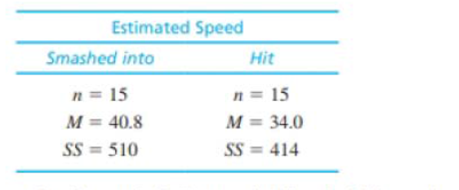Chapter 10, Problem 18PEssentials of Statistics for the B...

8th Edition
Frederick J Gravetter + 1 other
ISBN: 9781133956570

Solutions

Chapter
SectionEssentials of Statistics for the B...

8th Edition
Frederick J Gravetter + 1 other
ISBN: 9781133956570
Textbook Problem

In 1974, Loftus and Palmer conducted a classic study demonstrating how the language used to ask a question can influence eyewitness memory. In the study, college students watched a film of an automobile accident and then were asked questions about what they saw. One group was asked, “About how fast were the cars going when they smashed into each other?” Another group was asked the same question except the verb was changed to "hit'' instead of “smashed into.” The “smashed into” group reported significantly higher estimates of speed than the “hit” group. Suppose a researcher repeats this study with a sample of today’s college students and obtains the following results.a. Do the results indicate a significantly higher estimated speed for the “smashed into” group? Use a one-tailed test with α = .01.b. Compute the estimated value for Cohen's d to measure the size of the effect.c. Write a sentence demonstrating how the results of the hypothesis test and the measure of effect size would appear in n research report.

a.

To determine
Whether the result indicate a significantly higher estimated speed for the “smashed into” group using one-tailed test with α=0.01.

Explanation

Given info:

Two samples each with size 15 have means 40.8 and 34 respectively. The sum of squared difference (SS) is 510 and 414 respectively.

Calculations:

Step 1: Null hypothesis is H0:μ1μ20 and alternate hypothesis is H1:μ1μ2>0

Step 2: For the give sample, degrees of freedom is calculated as:

df=n1n22=15+152=28

For one-tailed test with α=0.01 and df=28, the t-table gives critical value (CV) equal to t=2.467

Step 3: t-statics is calculated using formula:

t=M1M2(μ1μ2)sM1M2

Pooled variance sp2:

sp2=SS1+SS2df1+df2=510+41414+14

b.

To determine

To find: The value of Cohen’s d.

c.

To determine
The outcome of study the way it would be reported.

Still sussing out bartleby?

Check out a sample textbook solution.

See a sample solution

The Solution to Your Study Problems

Bartleby provides explanations to thousands of textbook problems written by our experts, many with advanced degrees!

Get Started

Evaluate the integral. dxxx2+1

Calculus (MindTap Course List)

In Exercises 17-20, determine whether the point lies on the graph of the function. 18. (3, 3); f(x)=x+1x2+7+2

Applied Calculus for the Managerial, Life, and Social Sciences: A Brief Approach

Convert to whole or mixed numbers. 4.

Contemporary Mathematics for Business & Consumers

True or False: is monotonic.

Study Guide for Stewart's Single Variable Calculus: Early Transcendentals, 8th

True or False: Du f(a, b) = ∇(a, b) · u.

Study Guide for Stewart's Multivariable Calculus, 8th